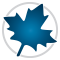Symbolic Computation - How Does Maple Compare? - Maplesoft# Maple

Compare: Symbolic Computation

## Benefits of Symbolic Computation

Symbolic computation lets you express and solve mathematical problems the way you think about mathematical problems-using variables, mathematical formulas, symbols such as π and ∞, and mathematical functions. Variables can remain as unknowns throughout your calculations. There is no need assign values to variables unless and until you want to and there is no need to introduce artificial approximations to get a calculation to work.

• Maple allows you to work with exact quantities such as fractions, radicals, and symbols, eliminating accumulated round-off errors. For example,can remain asthroughout your computation, resulting in an exact answer.
• Approximations can be computed to any precision that is required and are not restricted by hardware limitations. This "infinite precision" not only provides flexibility, but also makes it possible to avoid problems of catastrophic cancellation that lead to wildly inaccurate results at hardware precision.
• You can defer numeric approximations until they are needed, using symbolic parameters in your problem instead. The parameters are then carried through each stage in your analysis, making it easy to do parameter sweeps, optimize values, and study the behavior of the system.
• Symbolic computations allow you to obtain highly accurate results. They eliminate the need to simplify problems by hand and provide insight into your problem structure from which you can develop conjectures and conclusions about the behavior.

The symbolic capabilities of Maple have been under continuous development for over 25 years. Tapping into some of the best minds available, from both Maplesoft and renowned research institutes from around the world, Maple's symbolic engine has the breadth, depth, and performance that comes from years of dedicated development. Symbolic computation has been the core of Maplesoft's business since day one, and continues to be a key element in everything we do.

• Virtually every area of mathematics is covered, including calculus, integral and discrete transforms, linear algebra, statistics, signal processing, graph theory, differential geometry, linear programming, combinatorics, optimization, physics, and more.
• Maple's mathematical abilities include in-depth coverage of difficult problems. Examples include the following:

• Maple contains world-class differential equation solvers, including many new algorithms developed by Maple researchers.
• Because Maple combines symbolic and numeric techniques, Maple can apply hybrid solvers to differential-algebraic equations (DAEs). The symbolic preprocessing and order reduction means Maple can solve DAEs that other systems cannot.
• Maple's extensive collection of special functions, including Bessel functions and the LambertW function, means Maple can find closed-form solutions for a wide range of problems.
• Maple is not just a symbolic system. It provides completely integrated symbolic and numeric approaches. You can choose from a variety of approximate and exact techniques to suit your needs.
• Internally, Maple's solvers can also use a combination of symbolic and numeric techniques, allowing them to solve problems for which either approach alone would be insufficient.